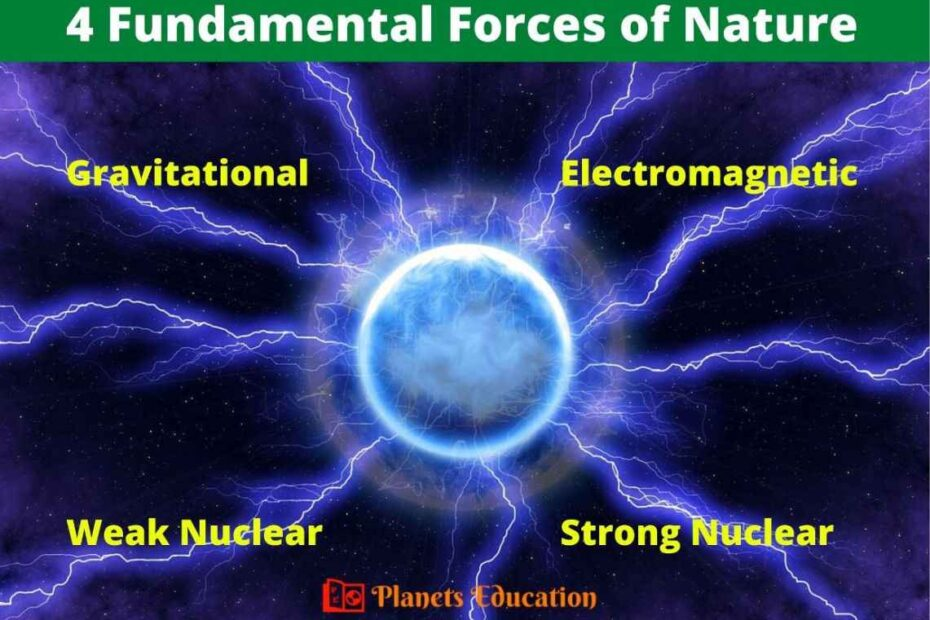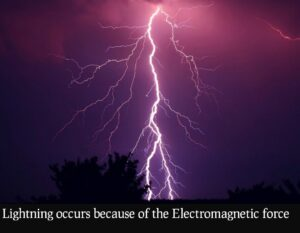# 4 Fundamental Forces of Nature in the UniverseThe 4 fundamental forces of nature also known as fundamental interactions are gravitational force, electromagnetic force, strong nuclear force, and weak nuclear force. These are the smallest force present in the universe and are not divisible into any basic forces.

The 4 fundamental forces of nature govern everything in the universe and hold atoms, planets, stars, solar systems, galaxies, and everything.

The effect of Gravitational force and electromagnetic force has a long range and it can be experienced in daily life. Whereas the effect of the strong force and weak force has a short range at a level of subatomic particles.

## List of fundamental forces

The 4 fundamental forces of nature are described by their carrier particles. Some of the elementary particles are responsible for carrying these forces and work as a force carriers. Here we have described the four fundamental forces and their force carrier particles in a table form:

 4 fundamental forces of nature Force carrier elementary particles Range of effect Gravitational Force Graviton (hypothetical) Infinity Electromagnetic Force Photons Infinity Weak Nuclear Force W & Z bosons 10-18 m Strong Nuclear Force Gluons 10-15 m
• According to the standard model of particle physics, the strong force is carried by gluons and it holds together the elementary quarks.
• The weak force acts on the nucleus of the atoms and is mediated by elementary W & Z bosons.
• The electromagnetic force is carried by photons and acts between electrically charged particles.
• Whereas the gravitational force is described by “Einstein’s general theory of relativity” as a space-time curvature and is carried by an elementary particle ‘graviton’ (graviton is a hypothetical particle- not discovered yet).

## 4 Fundamental Forces of Nature

The four fundamental forces of nature with their behavior in the universe are described here:

### 1. Gravitational force

Sir Issac Newton gave his ‘universal gravitation law’ in 1687 and according to this law “all bodies of the universe attract other bodies with a force called the gravitational force”. This force is directly proportional to their masses and inversely proportional to the distance between them.

F= G × m1× m2/r2

Where, G is the universal gravitational constant, m1 & m2 are the masses, and r is the distance between those two bodies.In 1915 Albert Einstein gave his general theory of relativity and according to this theory, gravity is described by space-time curvature.

If an object has more mass, it will create more curves in space-time and consequently will have more gravity.

• Fundamental gravitational force is the weakest force.
• It has an infinite range of effects.
• The gravitation is important for considering the force between distant objects.
• This is an attractive force and never repels.
• It is mostly considered for massive objects like planets, stars, and galaxies.
• This gravitational force matters most for observing the large-scale structure in the universe.
• Mass is the source of this fundamental force.

### 2. Electromagnetic force

Electromagnetic force or electromagnetism interaction acts between electrically charged particles. Electrically charged particles produce electromagnetic fields that are made of electrical fields and magnetic fields. The electromagnetic field is the reason to produces electromagnetic radiation or light

• Electromagnetic force has an infinite range of effects like gravitation.
• It is 2nd strongest fundamental force of nature in the universe after the strong nuclear force.
• The electromagnetic force is carried by elementary photons that travel at the speed of light.
• This force can be attractive and repulsive in nature. It shows a repulsion effect for like electrical charges, whereas an attraction effect for unlike charges. Electromagnetic force shows an attraction effect between the nucleus of an atom and electrons, hence it holds the atoms.
• The electromagnetic force has an infinite range of effects but it does not play a role in interstellar bodies like gravity. This is because the net electric charges are zero between these bodies. So this force is mostly considered for interaction between atoms.
• Electromagnetism interaction acts in the chemical bonding of the atoms.
• As this force have a macroscopic(infinite) range, so the distant effect of this force can also be seen in everyday life. For example, rainbows, lightning, and human-made devices like television, computers, etc### 3. Weak force

• The weak force is also called weak interaction or weak nuclear force. This force acts upon the subatomic particles of the atoms.
• Weak interaction force has a short-range (10-18 m) of effects as it is a nuclear phenomenon.
• The mediators of this force are elementary W & Z bosons.
• Radioactive decay or beta decay happens because of the phenomenon of weak forces in the nucleus.
• The combined effect of electromagnetic force and the weak force develops the term electroweak force.
• The weak nuclear force is the 2nd weakest fundamental force of nature after the gravitational force.
• Nuclear fission reaction is associated with weak nuclear force.

### 4. Strong force

• It is also known as a strong nuclear force or strong interaction. The strong nuclear force is responsible for holding elementary quarks together that form protons and neutrons. Hence this force is considered to hold ordinary matters in the universe
• Gluons are the force carrier elementary element for the fundamental strong nuclear force. As its name suggests ‘gluons’ act like a ‘glue’ and binds the quarks that subsequently form protons and neutrons.
• The strong interaction is the strongest fundamental force of nature. While comparing with the 2nd strongest electromagnetic force it is almost 137 times stronger. It is approximately 106 times stronger than a weak nuclear force and 1038 times stronger than a gravitational force.
• This force is unbelievably strong but shows effects in a very short range (10-15 m).
• The nuclear fusion reaction in the sun or stars is associated with a strong force.

These were the four fundamental forces of nature. With the help of these 4 forces of nature, our universe exists. The four fundamental forces run the system of the universe.

The 4 fundamental forces of the universe are gravitational, electromagnetic, strong nuclear, and weak nuclear forces. In which strong nuclear force is the strongest and gravitational force is the weakest.

Also Check:-

## 1 thought on “4 Fundamental Forces of Nature in the Universe”

1.In addition to these four forces, I believe a unified force at early history of our Universe, where all these four forces are equal and converge to a time frame of 10^-38 seconds after Big Bang Event at 10-^44 s, the Planck regime. The Energy scale, distance scale, time scale, and temperature scale are: 10^17 GeV, 10^-33 m, 10^-38 seconds and 10^30 Kelvin, respectively. The coupling constant appear to be that of the weak force? I call the fifth force as the Quantum Gravity Force or curvature of the spacetime.